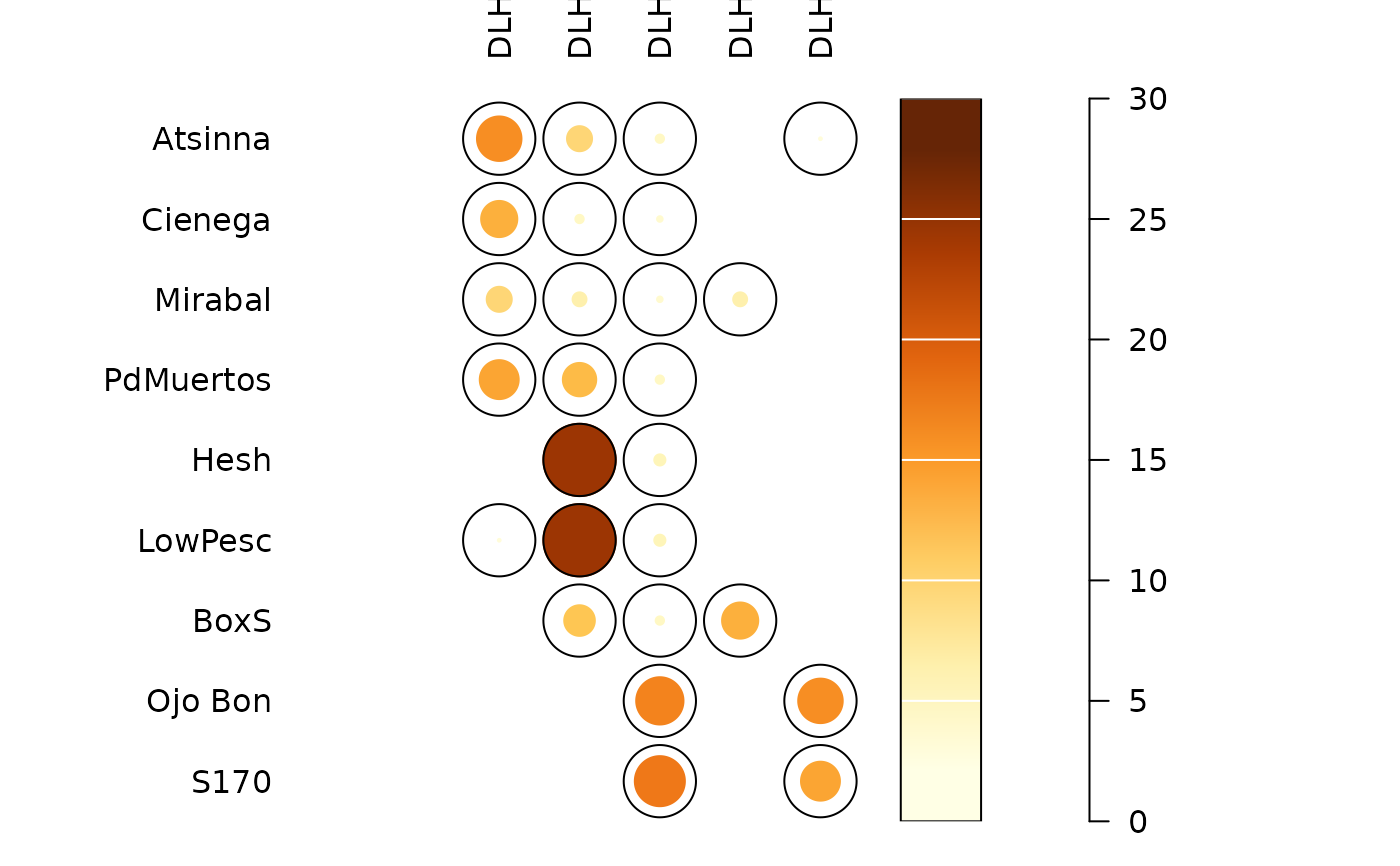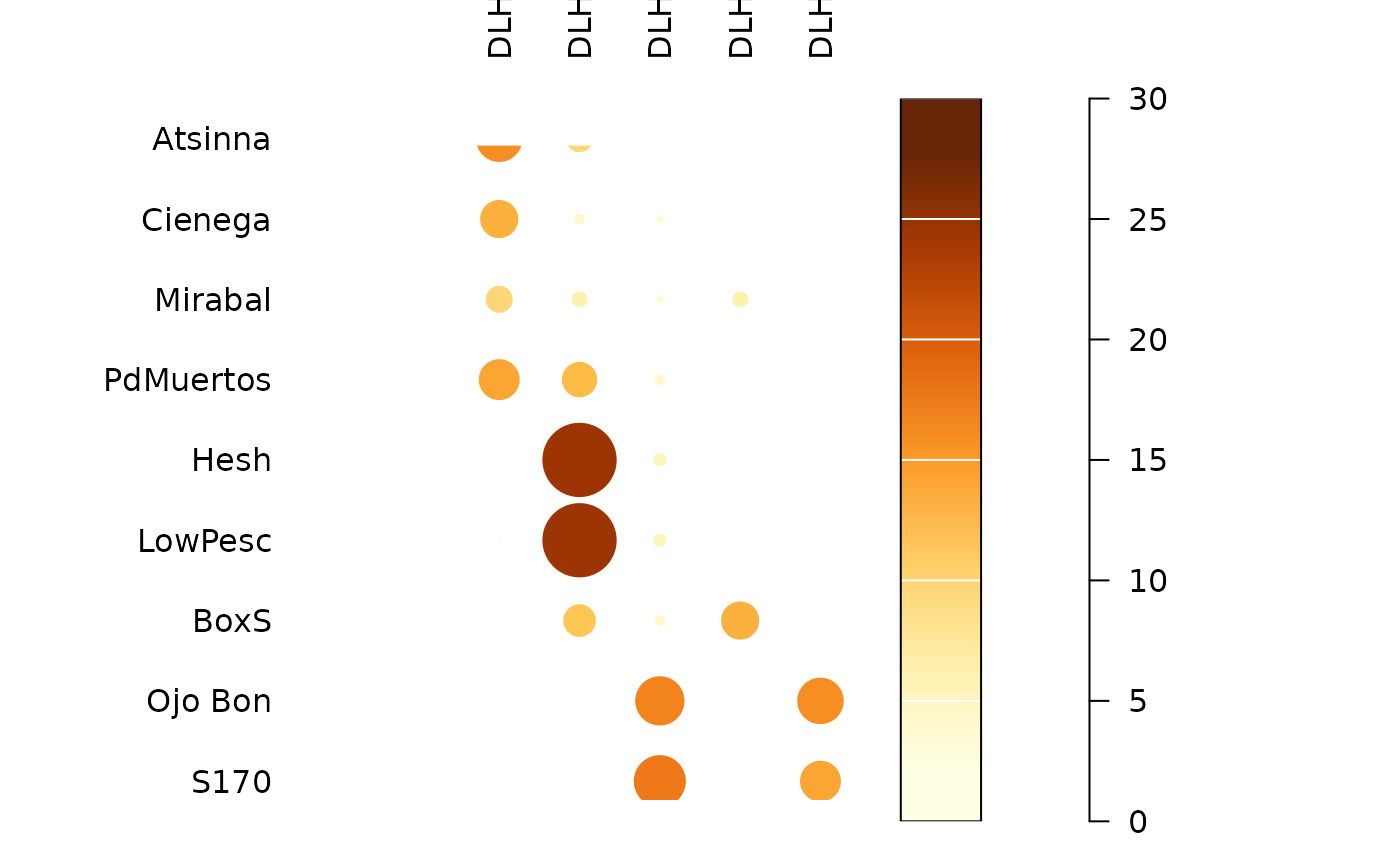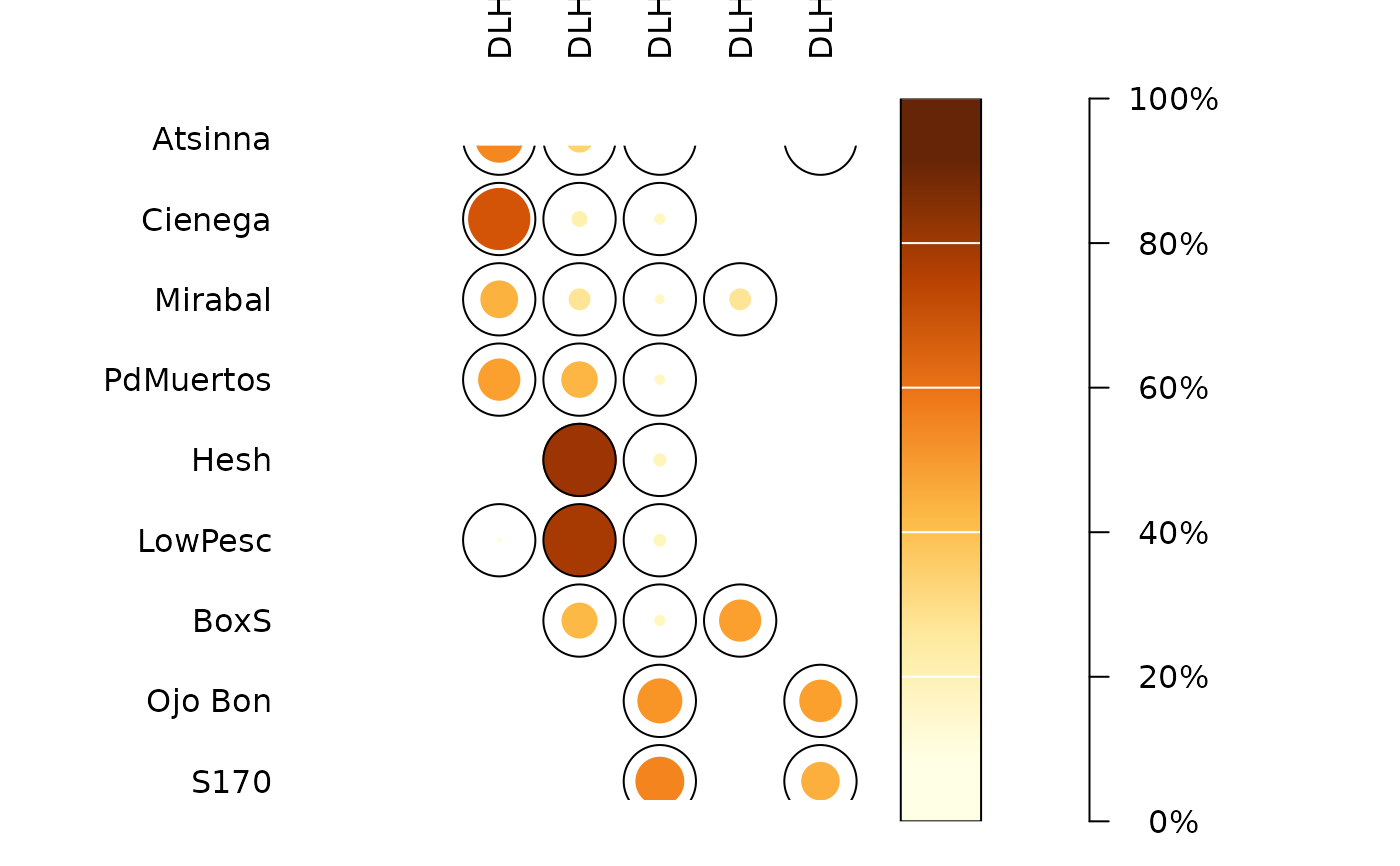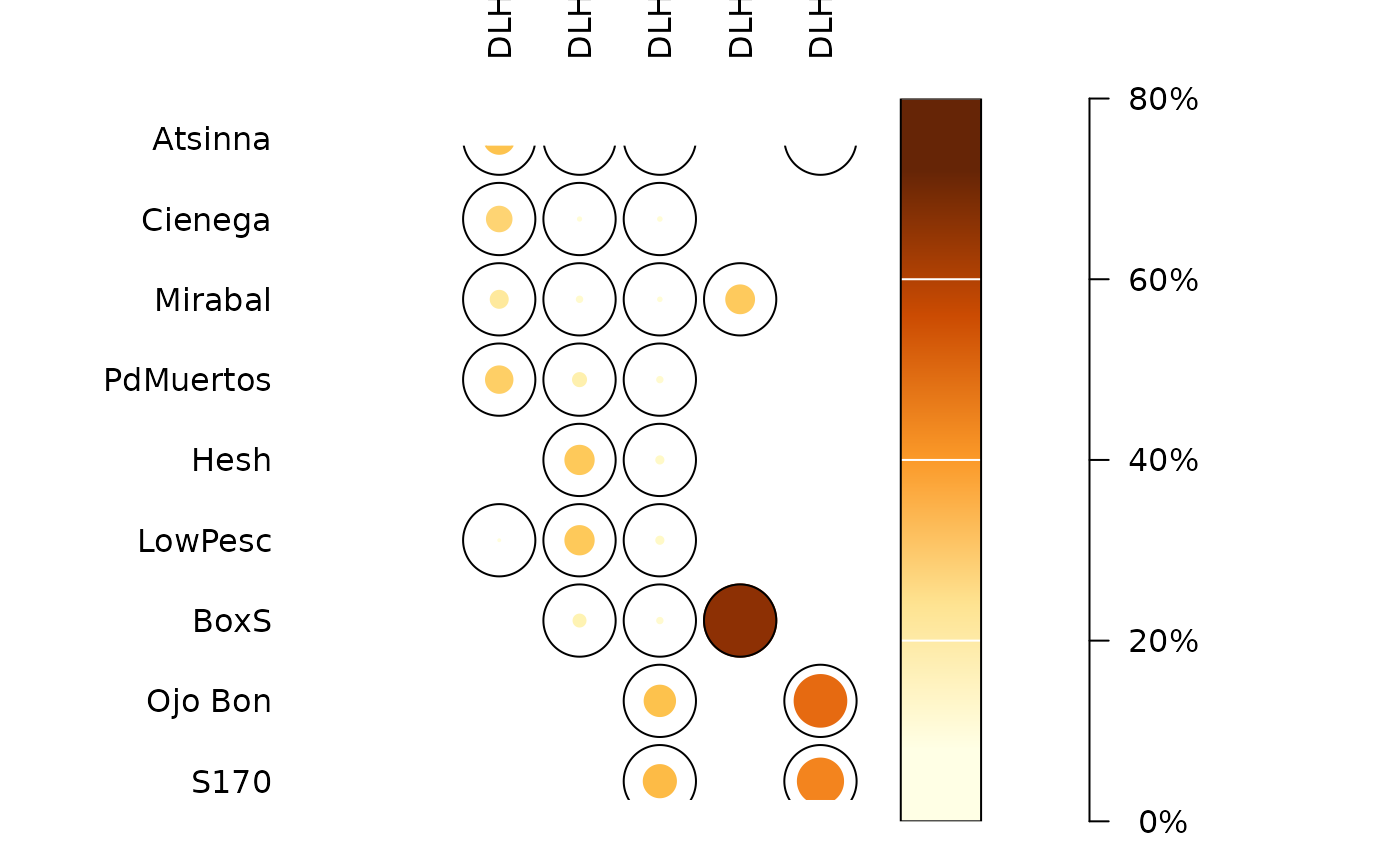Plots a spot matrix.

## Usage

plot_spot(object, ...)

# S4 method for matrix
plot_spot(
object,
type = c("ring", "plain"),
col = grDevices::hcl.colors(12, "YlOrBr", rev = TRUE),
diag = TRUE,
upper = TRUE,
lower = TRUE,
freq = FALSE,
margin = 1,
axes = TRUE,
legend = TRUE,
...
)

# S4 method for data.frame
plot_spot(
object,
type = c("ring", "plain"),
col = grDevices::hcl.colors(12, "YlOrBr", rev = TRUE),
diag = TRUE,
upper = TRUE,
lower = TRUE,
freq = FALSE,
margin = 1,
axes = TRUE,
legend = TRUE,
...
)

# S4 method for dist
plot_spot(
object,
type = c("ring", "plain"),
col = grDevices::hcl.colors(12, "YlOrBr", rev = TRUE),
diag = FALSE,
upper = FALSE,
lower = !upper,
axes = TRUE,
legend = TRUE,
...
)

## Arguments

object

A $$m \times p$$ numeric matrix or data.frame of count data (absolute frequencies giving the number of individuals for each category, i.e. a contingency table).

...

Currently not used.

type

A character string specifying the graph to be plotted. It must be one of "ring" (the default) or "plain". Any unambiguous substring can be given.

col

A vector of colors.

diag

A logical scalar indicating whether the diagonal of the matrix should be plotted. Only used if object is a symmetric matrix.

upper

A logical scalar indicating whether the upper triangle of the matrix should be plotted. Only used if object is a symmetric matrix.

lower

A logical scalar indicating whether the lower triangle of the matrix should be plotted. Only used if object is a symmetric matrix.

freq

A logical scalar indicating whether conditional proportions given margins should be used (i.e. entries of object, divided by the appropriate marginal sums).

margin

An integer vector giving the margins to split by: 1 indicates individuals/rows (the default), 2 indicates variables/columns. Only used if freq is TRUE.

axes

A logical scalar: should axes be drawn on the plot? It will omit labels where they would abut or overlap previously drawn labels.

legend

A logical scalar: should a legend be displayed?

## Value

plot_spot() is called it for its side-effects: it results in a graphic being displayed (invisibly returns object).

## Details

The spot matrix can be considered as a variant of the Bertin diagram where the data are first transformed to relative frequencies.

## Note

Adapted from Dan Gopstein's original idea.

Other plot methods: matrigraph(), plot_bertin(), plot_diceleraas(), plot_diversity, plot_ford(), plot_heatmap(), plot_rank(), plot_rarefaction, seriograph()

N. Frerebeau

## Examples

## Data from Huntley 2004, 2008
data("pueblo")

## Plot spot diagram of count data
plot_spot(pueblo, type = "ring")plot_spot(pueblo, type = "plain")## Plot conditional proportions
plot_spot(pueblo, freq = TRUE, margin = 1)plot_spot(pueblo, freq = TRUE, margin = 2)